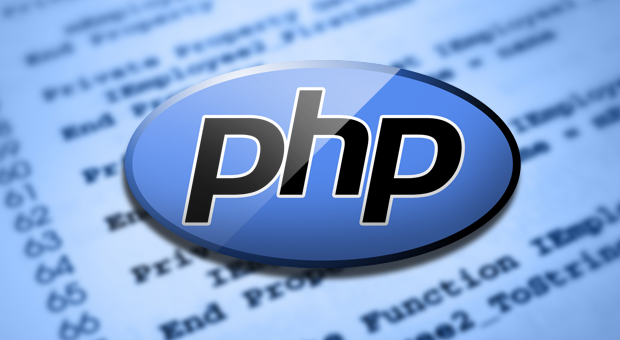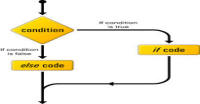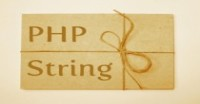# Introduction to PHP

Hello friends,

From last one week, I am getting a lot of requests from people for PHP. Here I am starting a PHP tutorial series. If you like these tutorial then please subscribe and share these tutorials.

Show all tutorials of this series till now

### What is PHP?

PHP stands for PHP: Hypertext Preprocessor. PHP is known as a server side scripting and interpreter language for web development. It means PHP is used on the server to render dynamic web pages. Dynamic web pages mean those pages which are created or modified during rendering from server to client. If you don't know what is server and what is client then open new tab in your browser and type  https://www.flowkl.com . Now you are client and from where the page is coming that is the server (web server).

### Installation:

You can easily install WAMP ( windows), LAMP (linux) or XAMMP (windows, mac and linux) according to your operating system. I am not telling you every step for installation because youtube already has a lot of videos of this purpose. I will prefer you to install XAMMP now and after learning basics, you should change for better understanding with servers. If you find some difficulty then ask me in comments. The php scripts run only in web browsers.

### Syntax:

Lets start coding.

```<?php
/*
author: Harish Kumar
website:www.flowkl.comm
version: 1.0
date: Mar 20 2015
*/
// this is single line comment
// comments are ignored by program/compiler
\$variable = 'variable';
// we use echo statement for printing
echo "string";
echo \$variable;
```

Copy and paste this code in a file named index.php inside your htdocs (on windows) or www (on linux) folder. Open this file in your browser (after starting server) you will get result as stringvariablename . Now try to understand the code.

### Syntax:

• PHP code is written in a file with extension .php
• PHP code is written between <?php and ?>
• Each assignment statement ends with semi-colon ';'
• single quotes and double quotes are equivalent (not equal) in PHP
• echo command is used to print the data in a web page.

The comments are the part of program which is not considered by compiler. These are the notes to remember/ understand the code later. There are two types of comments:

• Single line comment: These comments are short comments of size one line. These comments are started with '//'.
• Multiline comments: These are long size comment and may take more than one line. These comments are placed between /* and */

You can see both types of comments in the above example.

### Variables:

Variables are which let you store and manipulate data in your scripts. You can simply assume them like maths variables. A variable is simply a container that holds a certain value. Variables get their name because that certain value can change throughout the execution of the script. It’s this ability to contain changing values that make variables so useful.

As I have told before the variables name start with \$. Some valid examples of variable name are :

• \$simple
• \$_start_with_unserscore
• \$_includes_numbers_23
• \$_includes_UPPERCASE_2
• \$_23_it_is_valid

Note: you can not start a variable name with a number like \$2_invalid_variable_name.

### Data types:

Data types are the types of variables in PHP. In PHP, simple data types are integers (integer numbers), floats (decimal numbers), string (text line) and boolean (true or false). There are some more data types like objects and array, but we will study later about them.
Note: If you have strong type language background like C, C++, Java, C# etc. then you should note that PHP is weak/ loosly typing language, i.e. you don't have to tell which data type is stored in the variable.

### Constants:

You can also define fixed value variables. These variables can not be changed after defining once.

```<?php
/*  author: Harish Kumar
website: www.flowkl.com
version: 1.0
date: Mar 20 2015
*/
// this is single line comment
\$variable = 'variablename';
echo "string";
echo \$variable;
define( "MY_CONSTANT", "19" );
define('PI', 3.14);
echo PI;
echo MY_CONSTANT;
```

Here PI and MY_CONSTANT are fixed variables.

### Operators:

PHP has many types of operators. Most :

1. Arithmetic
2. Assignment
3. Bitwise
4. Comparison
5. Incrementing/Decrementing
6. String

Arithmetic operators are your maths operators. These are +, -, *, / and %

Example:

\$a = 4 + 5; // + is an arithmetic operator

Note: there is a difference between '/' and '%' operator. '/' is divide operator while % is modulus operator.

Example: 8 / 5 = 1.6 ( 8 = 5*1 + 3) while 8 % 5 = 3 ( 3 is reminder)

Assignment operator assigns values to variables.

Example: \$a = 4; ('=' is an operator)

Bitwise operators performs operations on individual bits in an integer. Bitwise operators are & (and), | (or), << (left sift) , >> (right sift), ^ (XOR) and ~ (NOR).

Example: 14 & 3 = 2 (00001110 & 00000011 = 00000010 these numbers are in binary).

Comparison operators are used to compare one operand to another.

Incrementing/ decrementing operators are for increasing/decreasing the value of the variable by one unit.

Example:

++\$x; // Adds one to \$x and then returns the result
\$x++; // Returns \$x and then adds one to it
–-\$x; // Subtracts one from \$x and then returns the result
\$x–-; // Returns \$x and then subtracts one from it

String operatior is use for concat two srings or variables. For example use following code:

```<?php
\$a = 4;
\$q = 5;
\$c = \$a.\$q; // \$a and \$q are joined as string
echo \$c;
\$n = 'myname';
echo "string  ".\$n.' \$n inserted'; // joining three things
```

### Exercise:

1. Try to define and print your own variables
2. Try to use various operators on your variables and print the result

In next tutorial we will study about conditional statements in PHP. If you like it then please share it with your friend otherwise give your valuable feedback to improve this as well as future tutorial to help other people.

Don't forget to Subscribe for latest feeds.

next tutorialHarish, a fullstack developer at www.lyflink.com with five year experience in full stack web and mobile development, spends most of his time on coding, reading, analysing and curiously following businesses environments. He is a non-graduate alumni from IIT Roorkee, Computer Science and frequently writes on both technical and non-technical topics.

### Related ArticlesPHP stands for PHP: Hypertext Preprocessor. PHP is known as a server side scripting and interpreter language for web ...
##### Introduction to PHPIn this tutorial, we will study about conditional statements (if else statements and switch statements). These statem...
##### Conditional statements in phpIn this tutorial, we will study about strings and operations upon strings. Due to long length of this section, I have...

### Complete Python Bootcamp: Go from zero to hero in Python 3### The Complete Web Developer Course - Build 25 Websites